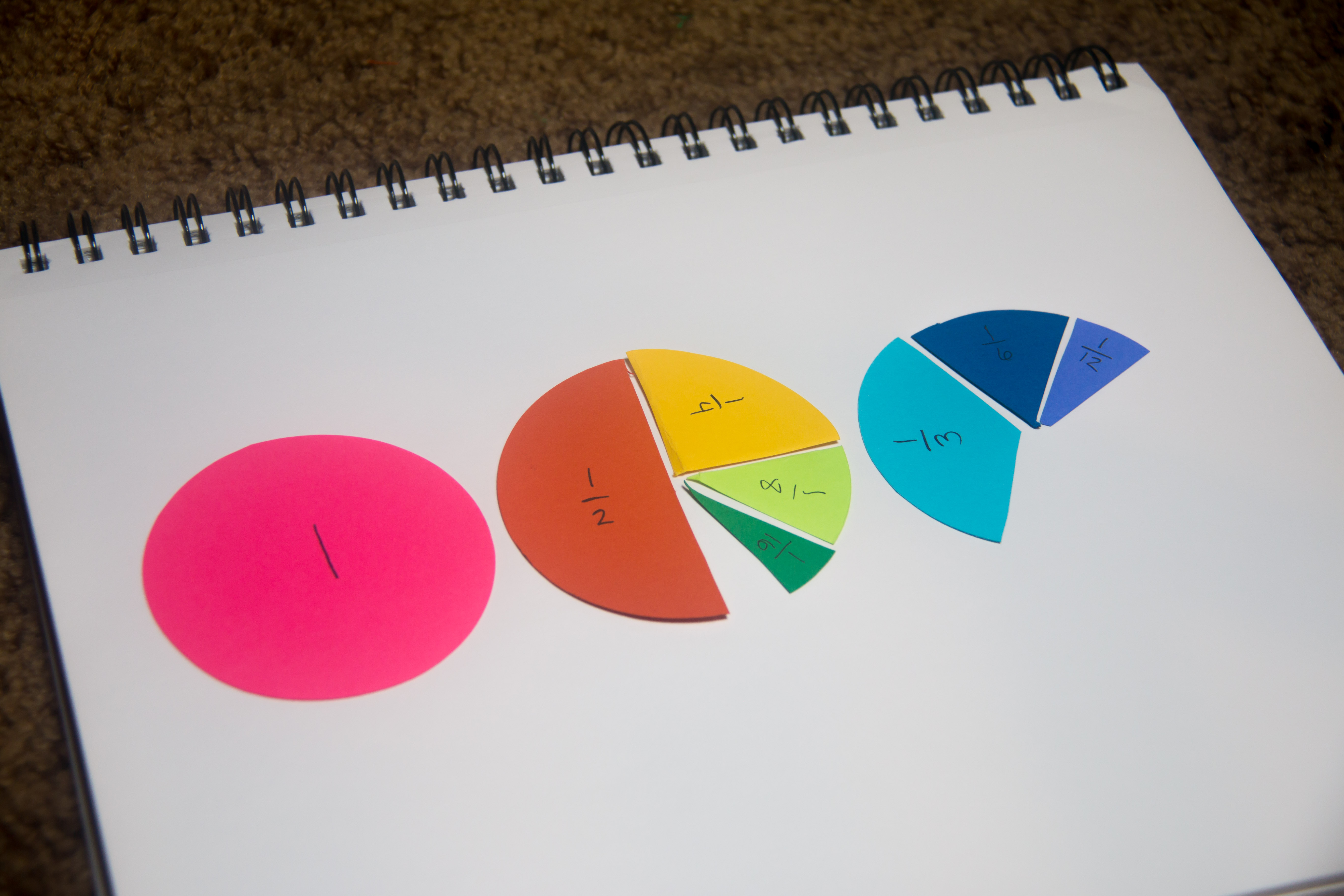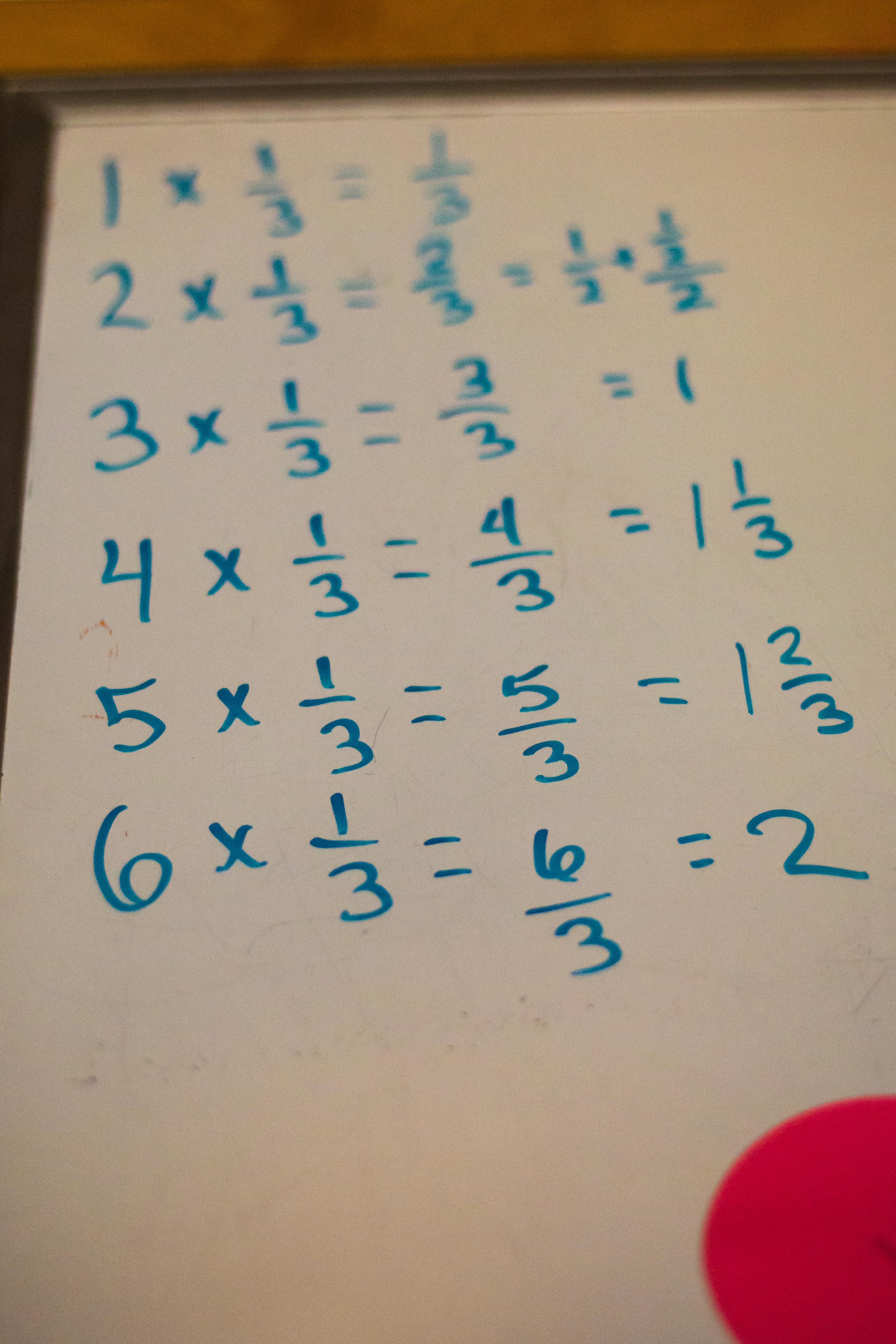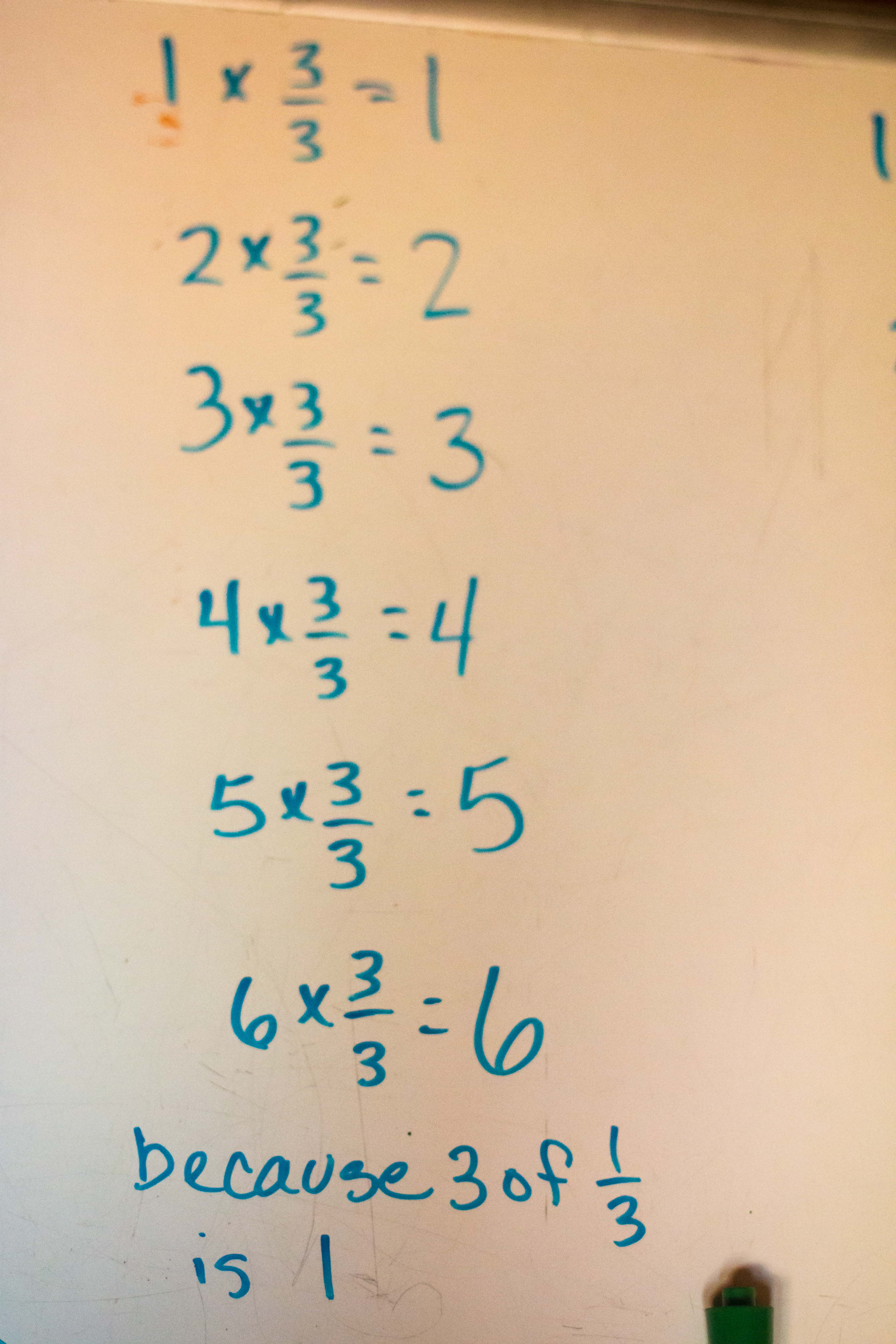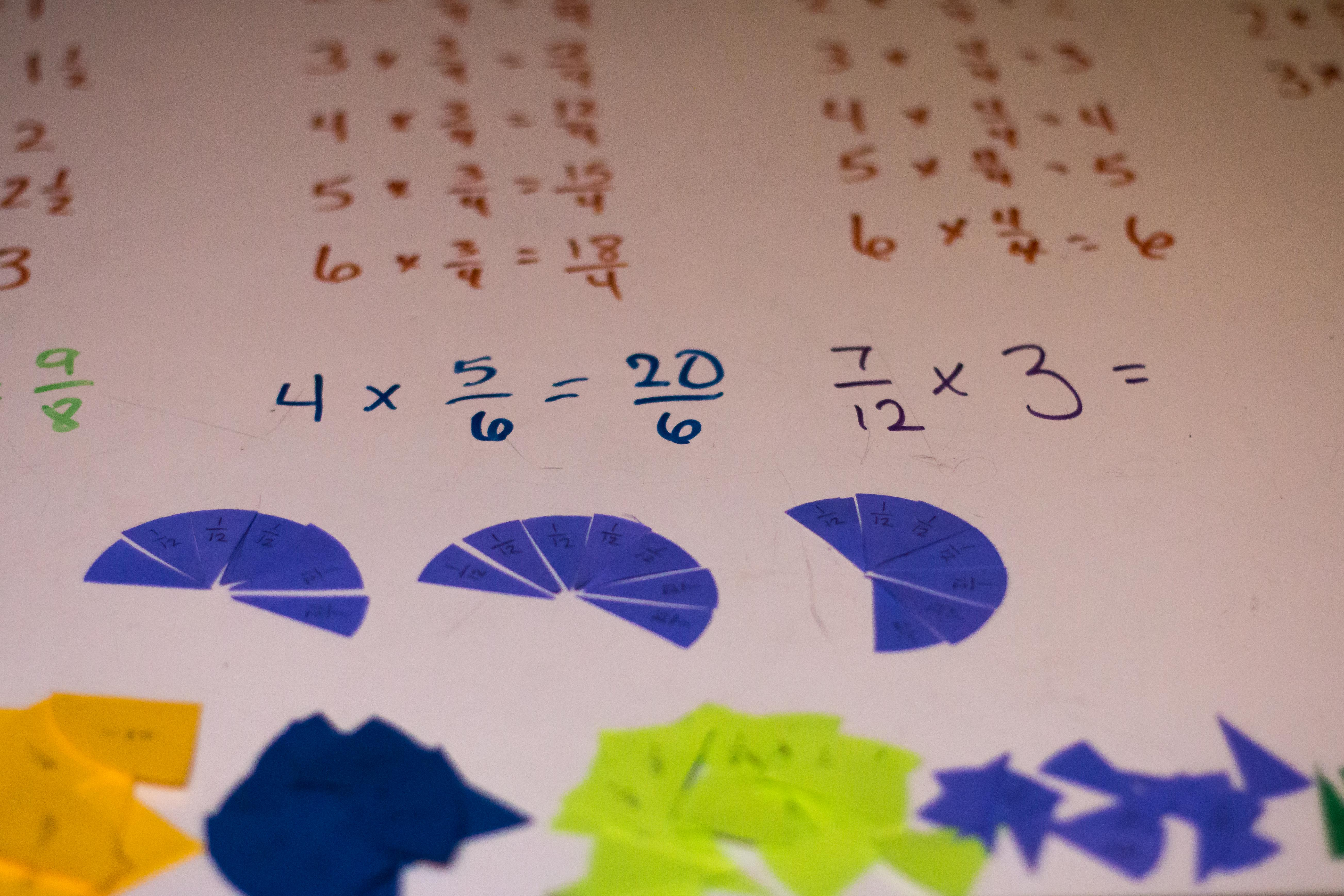# Fractions-Circles

This is a math series follows our unit for introduction to fractions in grade 3.  This is one of several, and I’m combining several days into one post. This part of the unit all involves circles.fractions cut from circles; finding halves of half, forth, eighth, third, and sixths.

## Day 1

We opened our fractions unit with cutting circles. I precut 8 different colors of circles, 3 of each (so there will be enough for adding, mix fractions, and finding equivalent fractions. We started first by halving a circle. Most children understand what halving is. They have had to share enough with a sibling or friend that this particular fraction is very real to them. I explained how we write a fraction with a numerator being the number of pieces we have, and denominator being the number the item is divided into. Then we went onto halving a half to see how many pieces we get. That also came fairly quickly because we’ve talked about a quarter of something before now. Then we halved the forth to obtain our eighths and then halved our eighths to get our sixteenths.finding 1/2’s of fractions using circles including half, forth, eighth, sixteenth, third, sixth, and twelfth

I really enjoy math, and one of the things that I love about math is the patterns. So when doing math with my children, I will lead them through several processes and then ask them what they notice. When first starting this process, sometimes it takes an adult jump start the process. “I notice…” and see what the child says. Here you will notice that each time we half a fraction, it’s denominator doubles. She noticed it too. From there when we went on to halving the thirds, she was able to predict that we would get sixths, and then the twelfths. Without further manipulatives, she could tell me what 1/2 of 1/5 would be and 1/2 of 1/7.

## Day 2

Each day before we begin the main lesson, I plan to review what we “discovered” the day before. We did this today talking about halving our halves and then fourths and so forth, and cutting out all of our fractions. From then we moved onto our main lesson.

### Sorting

First we began with sorting our fractions of circles by size from the smallest to the largest. Then we moved on to sorting by the denominator. At this point I prompted her to notice what was happening. She noticed two things: First that they were the same order, but that they went backwards, and the second that as the denominator gets bigger, there are more pieces. Then I said, “I wonder where 1/5 would fit?” If you remember from day 1, we halved halves from 1 to 1/16 and then from 1/3 to 1/12, but we did not do tenths, fifths, sevenths, or ninths. It was relatively easy for her to see that 1/5 would fit between 1/4 and 1/6 and to estimate the size of a 1/5 pie slice.sorting fractions by size and by size of denominator using circles

### Equivalent fractions

At this point I was trying to decide which direction that I wanted to lead her – equivalent fractions, or sequencing (i.e. 1/2, 1, 1 1/2, 2…) when she mentioned that a few of the fractions were the same, and I asked what she meant. She was noticing equivalent fractions, so we went in that direction.
First I had her find all the equivalents for 1/2. As we went along, first by using the size of the fraction to compare, she quickly picked up the pattern and she was able to find the fraction just from the pattern. Notice that she was able to do this for thirds, even though we don’t have a comparable fraction cut for 1/2. From here I pulled out our 100 chart (tutorial for making them here) using our multiplication side to show that you can find equivalent fractions from using our multiplication chart.
I asked if she thought this would work for the other rows, and we pulled them out using the fractions that we had to compare to see if it did work for each row. You will see that it does, and after 1/2, 1/4 has the most equivalent fractions represented in the multiplication chart from the fractions that we cut earlier. I felt like this was enough for the day, and I wanted to end the main lesson on a high note, so we stopped here.

## Day 3

We started our number line.  You can find that here.

## Day 4

### Adding and Subtracting Fractions

Today was raining and dreary and so we returned to our circles.  We focused primarily on adding and subtracting fractions.  I began with our biggest fraction-the half- and we added those.I began with 1/2 + 1/2 and then 1/2+1/2+1/2.  Then we moved onto to more complicated things like 3/2 + 1/2. Then we “reduced” by finding the mixed fraction.  We repeated this with thirds and then fourths.

After fourths we would pause to “notice.”  Though I saw that she understood that the fractions were added just as whole numbers, she didn’t express that.  What she did express is that she noticed that it takes the same number of fractions as the denominator to make one, i.e. it takes 8 of 1/8 to make one. I thought this was a significant observation.  I noted this in her math journal, and we moved on.

From here we moved onto subtraction.  We worked with the smaller fractions since there were more of them to work with.  I took a turn giving her a problem that she would work out and then find all the equivalents, and then she would give one to me.  After doing this a few times, we stopped to make more observations. I noted that when we added fractions, the numerator changed, but the denominator did not.  We have not added or subtracted fractions with different denominators. If it comes up in our play, I’ll go with it. Otherwise I’ll wait until next year.

## Day 5

### Multiplying fractions and whole numbers

In exploring the concept of multiplying fractions, instead of just showing her how this works with manipulative and numbers, I make a list of (boring, yet) systematic calculations.  We started with our largest fraction 1/2 and worked through our circle fractions of 1/3, and then 1/4 until a pattern of fractions in general began to emerge for her and also making sure to keep her attention. I will say, seeing all the calculations listed out was a little overwhelming to her, but I assured her once she found the pattern, it would go very quickly.

We begin with a column multiplying each whole number by our fraction.  In our example below, the fraction is thirds.  In doing this, she quick sees a pattern and is able to finish the rest of the problems with ease.Then we move on to multiplying by 2 of our fractions, in this example 2/3 by the whole numbers 1 through 6. Again, a pattern begins to emerge.  She noticed that this time we are skip counting by 2’s with a third as the unit.From here we move onto 3/3.  As you may have noted earlier, she had already noticed that it takes the number of fractions as the denominator to make a whole. In this example 3 of thirds to make one, so it came very quickly to her that we were just multiplying by 1.An in our last column we multiplied with a fraction greater than 1, in this case 4/3. She noticed that here are we skip counting by 4’s or multiplying by 4 and the whole number in the units of thirds.After we did this systematic calculations with halves, thirds, and fourths, we moved onto guessing and figuring out calculations with other fractions.  We did at least one calculation with each of our other fractions: sixths, eighths, twelfths, and sixteenths. After calculating, we would use our fractions to make sure our answer was correct.  Note in the picture of the dark blue sixths, that we did not have enough paper fractions and we move into drawing the figure.  This is a great tool to use for calculations through even high school and college. Many, like me, find visual representations very helpful.## Day 6

Today was spent exploring division of whole numbers by fractions. We started methodically like the other exposures beginning with “1.”  We did 1 ÷ 1/2, then 1 ÷ 1/3, then 1 ÷ 1/4, and so forth with all the fractions that we had.  Then we practiced on a few that we did not have like 1/9.  From here we just played and explored what would fit into our other fractions and how many of them would. For instance who many 1/8 would fit into 1/2.  This is another way of exploring division.  Our exploration of division was short and sweet.  The big pattern of being able to divide by multiplying by the reciprocal is a larger one that she is not quite ready for. We’ll explore that maybe next year.

To continue with our fractions explorations, read the post Fractions on a Number Line

Just a note: I participate in Affiliates Programs. When you use the links above, your cost is the same, but I receive a very small commission from your purchase. I use this commission to fund my website to keep it ad free. I want you to experience my website without being bombarded with ads. Thanks for your support. For more information on my relationships see my Policies and Disclosures.